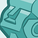# New to Qlik Sense

If you’re new to Qlik Sense, start with this Discussion Board and get up-to-speed quickly.

Announcements
Qlik Cloud Maintenance is scheduled between March 27-30. Visit Qlik Cloud Status page for more details.
cancel
Showing results for
Did you mean:Contributor II

## YTD, FYTD Calculations

Hi

I am learning Qlik Sense and I want to make a date comparison of YTD, MTD, FYTD

can someone please guide me on how to write a set expression step by step and write a code for YTD, FYTD, and MTD till the date selected in the date picker, not today's day

Labels (4)

• ### Variables

2 Solutions

Accepted SolutionsChampion III

As below

YTD = sum({<Date={">=\$(=Date(YearStart(max(Date)),'YourDateFormat'))<=\$(=Date(Max(Date),'YourDateFormat')"}>}Amount)

MTD = sum({<Date={">=\$(=Date(MonthStart(max(Date)),'YourDateFormat'))<=\$(=Date(Max(Date),'YourDateFormat')"}>}Amount)

FYTD = sum({<Date={">=\$(=Date(YearStart(max({1}Date)),'YourDateFormat'))<=\$(=Date(Max({1}Date),'YourDateFormat')"}>}Amount)

Vineeth Pujari
If a post helps to resolve your issue, please accept it as a Solution.Champion III

so FYTD is fiscal ytd I assumed it was Full Year ToDate;

FYTD  = sum({<Date={">=\$(=Date(YearStart(max(Date),0,4),'YourDateFormat'))<=\$(=Date(Max(Date),'YourDateFormat')"}>}Amount)

The above assumes April as the first month of the fiscal year, you can modify it as needed

refer below on how to generate a fiscal calendar

https://community.qlik.com/t5/QlikView-Documents/Fiscal-and-Standard-Calendar-generation/ta-p/148082...

Vineeth Pujari
If a post helps to resolve your issue, please accept it as a Solution.
5 RepliesChampion III

As below

YTD = sum({<Date={">=\$(=Date(YearStart(max(Date)),'YourDateFormat'))<=\$(=Date(Max(Date),'YourDateFormat')"}>}Amount)

MTD = sum({<Date={">=\$(=Date(MonthStart(max(Date)),'YourDateFormat'))<=\$(=Date(Max(Date),'YourDateFormat')"}>}Amount)

FYTD = sum({<Date={">=\$(=Date(YearStart(max({1}Date)),'YourDateFormat'))<=\$(=Date(Max({1}Date),'YourDateFormat')"}>}Amount)

Vineeth Pujari
If a post helps to resolve your issue, please accept it as a Solution.Contributor II
Author

Thank you so much for this solution it works fine against YTD, MTD

but I want to understand how the Fiscal year to date is working?Champion III

so FYTD is fiscal ytd I assumed it was Full Year ToDate;

FYTD  = sum({<Date={">=\$(=Date(YearStart(max(Date),0,4),'YourDateFormat'))<=\$(=Date(Max(Date),'YourDateFormat')"}>}Amount)

The above assumes April as the first month of the fiscal year, you can modify it as needed

refer below on how to generate a fiscal calendar

https://community.qlik.com/t5/QlikView-Documents/Fiscal-and-Standard-Calendar-generation/ta-p/148082...

Vineeth Pujari
If a post helps to resolve your issue, please accept it as a Solution.Contributor II
Author

Thank you So much its working fine nowChampion III

happy to help

Vineeth Pujari
If a post helps to resolve your issue, please accept it as a Solution.Tags
Community Browser